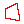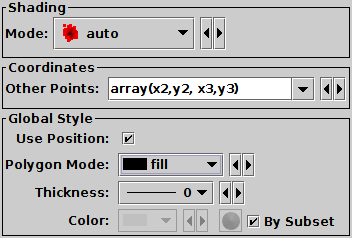Next Previous Up Contents
Next: Line Form
Up: Plot Forms
Previous: SkyCorr Form

#### A.4.5.11 Polygon Form

The Polygon () form plots filled or outlined polygons with an arbitrary number of vertices. The first vertex is given in the Position Layer Control's Position tab, and the others are given in the Coordinates box within the Form tab.Example Polygon plotPolygon form configuration panel

The configuration options are:

See Appendix A.4.6.
Other Points
Array of coordinates giving the polygon vertices excluding the first vertex, which is given by the main Position. These coordinates are given as an interleaved array, e.g. `(x2,y2, x3,y3, x4,y4)`. Expression language functions including `array(a,b,c,...)` from the Arrays class, and `parseDoubles(txt)` from the Conversions class, can be useful here. Although the first coordinate pair is supposed to be given in the Position tab and not in this array, if it's more convenient to repeat the first pair here it doesn't usually affect the plot's appearance.
Use Position
Determines whether the basic positional coordinates for the plotted row are included as one of the polygon vertices or not. The polygon has N+1 vertices if this value is set, or N if it is not, where N is the number of vertices supplied by the Other Points array. If unset, the reference position is ignored for the purposes of drawing the polygon.
Polygon Mode
Defines how the polygon is drawn. Options are
• `outline`: draws a line round the outside of the polygon
• `fill`: fills the interior of the polygon
• `cross`: draws a line round the outside of the polygon and lines between all the vertices
Thickness
Controls the line thickness used when drawing polygons. Zero, the default value, means a 1-pixel-wide line is used. Larger values make drawn lines thicker, but note changing this value will not affect all shapes, for instance filled polygons contain no line drawings.

Next Previous Up Contents
Next: Line Form
Up: Plot Forms
Previous: SkyCorr Form

TOPCAT - Tool for OPerations on Catalogues And Tables